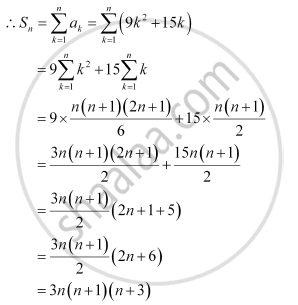CBSE (Arts) Class 11CBSE
Share
Notifications

View all notifications
Books Shortlist
Your shortlist is empty

# Find the Sum to N Terms of the Series 3 × 8 + 6 × 11 + 9 × 14 +… - CBSE (Arts) Class 11 - Mathematics

Login
Create free account

Forgot password?
ConceptSum to N Terms of Special Series

#### Question

Find the sum to n terms of the series 3 × 8 + 6 × 11 + 9 × 14 +…

#### Solution

The given series is 3 × 8 + 6 × 11 + 9 × 14 + …

a= (nth term of 3, 6, 9 …) × (nth term of 8, 11, 14, …)

= (3n) (3n + 5)

= 9n2 + 15nIs there an error in this question or solution?

#### APPEARS IN

NCERT Solution for Mathematics Textbook for Class 11 (2018 to Current)
Chapter 9: Sequences and Series
Q: 6 | Page no. 196
Solution Find the Sum to N Terms of the Series 3 × 8 + 6 × 11 + 9 × 14 +… Concept: Sum to N Terms of Special Series.
S